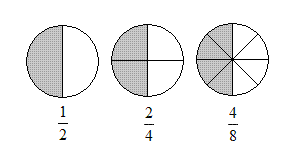# Equivalent Fractions

Two fractions are equivalent if they represent the same value.

For example, the figure below shows that $\frac{1}{2},\frac{2}{4}$ and $\frac{4}{8}$ are all equivalent fractions -- in each figure, the shaded portion represents half of the whole circle.In general, if you multiply the numerator and denominator of a fraction by the same (nonzero) number, you get a fraction equivalent to the one you started with. This is because when you multiply by a fraction like $\frac{2}{2}$ or $\frac{7}{7}$ , you're really just multiplying by $1$ -- and multiplying by $1$ always gives you the same number you started with. (Even if it looks different.)

For example:

$\frac{2}{5}=\frac{2\text{\hspace{0.17em}}\cdot \text{\hspace{0.17em}}7}{5\text{\hspace{0.17em}}\cdot \text{\hspace{0.17em}}7}=\frac{14}{35}$